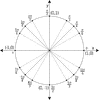# Unit Circle Labeled At Special AnglesIllustration of a unit circle (circle with a radius of 1) superimposed on the coordinate plane with the x- and y-axes indicated. All quadrantal angles and angles that have reference angles of 30°, 45°, and 60° are given in radian measure in terms of pi. At each quadrantal angle, the coordinates are given. These coordinates can be used to find the six trigonometric values/ratios. The x-coordinate is the value of cosine at the given angle and the y-coordinate is the value of sine. From those ratios, the other 4 trigonometric values can be calculated.

Unit Circles

### Source

Florida Center for Instructional Technology Clipart ETC (Tampa: University of South Florida, 2007)

EPS (vector)

431.1 KiB

TIFF (full resolution)

2971×3000, 312.8 KiB

Large GIF

1014×1024, 40.5 KiB

Medium GIF

633×640, 23.8 KiB

Small GIF

316×320, 9.8 KiB